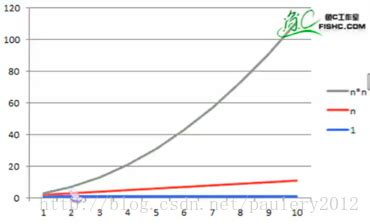# 数据结构第二章 算法

1. 1:算法：
2. 2:算法的五个基本特性：输入、输出、有穷性、确定性和可行性
3. 3:算法设计的要求：正确性、可读性、健壮性、时间效率高和存储量低
4. 4:算法效率的度量方法
5. 4.1:O(1)<O(log n)<O(n)<O(n log n)<O(n^2)<O(n^3)<O(2^n)<O(n!)<O(n^n)

## 算法：

#### 算法是解决特定问题求解步骤的描述，在计算机中表现为指令的有限序列，并且每条指令表示一个或多个操作

###### 用程序实现如下：
``````int i,sum = 0,n = 100;
sum = (1+n)*n/2;
printf("%d",sum);
``````

## 算法的五个基本特性：输入、输出、有穷性、确定性和可行性

• 输入输出：算法具有零个或多个输入，至少有一个或多个输出
• 有穷性：指算法在执行有限的步骤之后，自动结束而不会出现无限循环，并且每一个步骤在可接受的时间内完成
• 确定性：算法的每一步骤都具有确定的含义，不会出现二义性
• 可行性：算法的每一步都必须是可行的，每一步都能通过执行有限次数完成

## 算法设计的要求：正确性、可读性、健壮性、时间效率高和存储量低

• 正确性：算法的正确性是指算法至少应该具有输入、输出和加工处理无歧义性、能正确反映问题的需求、能够得到问题的正确答案
• 可读性：要便于阅读、理解和交流
• 健壮性：当输入数据不合法时，算法也能做出相关处理，而不是产生异常或莫名其妙的结果
• 时间效率高和存储量低：设计算法应该尽量满足时间效率高和存储量低的需求，用最少的存储空间实现算法功能

## 算法效率的度量方法

``````/*第一种算法*/
int i,sum = 0,n = 100; /*执行1次*/
for(i = 1;i < = n;i++) /*执行n+1次*/
{
sum = sum+i;  /*执行n次*/
}
printf("%d",sum);  /*执行1次*/
``````
``````/*第二种算法*/
int i,sum = 0,n = 100;/*执行1次*/
sum = (1+n)*n/2;/*执行1次*/
printf("%d",sum);/*执行1次*/
``````# 算法时间复杂度

#### 推导大O阶：

• 用常数1取代运行时间中的所有加法常数
• 在修改后的运行次数函数中，只保留最高阶项
• 如果最高阶项存在且不是1，则去除与这个项相乘的常数

##### 上面例子中两个算法的时间复杂度分别是O(n)和O(1)。

O(1) 常数阶
O(n) 线性阶
O(n^2) 平方阶
O(log n) 对数阶
O(n log n) n log2 n阶
O(n^3) 立方阶
O(z^n) 指数阶

Title - Artist
0:00